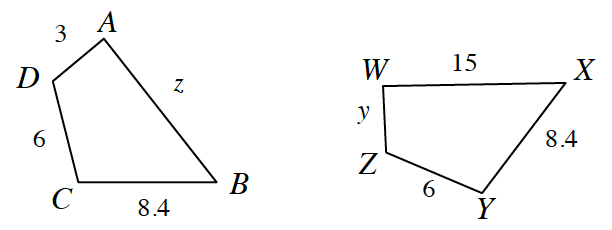### Home > CC4 > Chapter 8 > Lesson 8.1.1 > Problem8-18

8-18.

Use tracing paper and a series of rigid transformations to determine if these two polygons are congruent. If possible, find the lengths of $z$ and $y$. Homework Help ✎What two transformations are needed to move $ABCD$ onto $WXYZ$?

To find $z$ and $y$, divide or multiply by the zoom factor.

$y=3$